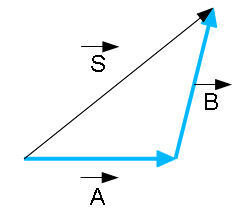# Prove the law of cosines.

## Homework Statement

Prove the law of cosines: If v and w are vectors in R^2, then

|v-w|^2 = |v|^2 + |w|^2 - 2|v||w|cosθ

where θ is the angle between v and w.

2. Homework Equations /info

|x| refers to length, and not absolute value (If that doesn't make any sense then I must be wrong, but our prof. insisted on only using single lines to define a value as 'length', which can be confusing at times).

## The Attempt at a Solution

I got blindsided by this question (missed the lecture..). Even just a starting point, or even an online resource would be greatly appreciated, and to go as far fully providing me with the solution would be extremely helpful (but I suppose possibly detrimental).

Also, the rest of the assignment deals with orthogonal projections, and cross products, as well as distances from points to planes, and lines - do these sort of questions involve the usage of the above law?

Do you mean you want to prove the following:CompuChip
Homework Helper
Assuming you know the explicit form of the dot product ($\vec a \cdot \vec b = |\vec a| |\vec b| \cos\theta$ with $\theta$ the angle between them) you can just write it out using the properties of the dot product:
$$|v - w|^2 = (v - w) \cdot (v - w) = \cdots$$

Alright,

So I get:

v2 - 2vw +w2 = v2 + w2 - 2vwcosθ

Then:

-2vw = -2vwcosθ

so for that to be true cosθ needs to equal 1, meaning theta is 0.

..now what?

CompuChip
Homework Helper
Sorry, what?
Where is the left hand side coming from?
And what does "2vwcosθ" mean? v and w are vectors, vw is not a valid product.
Please try to be a bit more precise in your notation, it is important here!

Right sorry,

So doing the dot product of the LHS gives us:

|v|2 + |w|2

Then, we substract the lengths |v|2 + |w|2 of the RHS from the lengths |v|2 + |w|2 of the LHS , which leaves

0 = 2 |v| |w| cosθ

I'm still confused as to where this is going. I really am sorry for being so clueless on this matter.

Hold on.. If 0 = 2 |v| |w| cos (theta) then that means cos of theta has to equal 0 (so cos of 2 over pi) meaning the angle is 90 degrees, meaning the two vectors are perpendicular.

Right?

CompuChip
Homework Helper
Yes, I think you are missing my point.
The equality I wrote,
$$|v - w|^2 = (v - w) \cdot (v - w),$$
is the definition of |v - w|, because the length of a vector is the square of the dot product with itself.
You can then work out the dot product, but note that wherever you get v . v, you don't really get v2, but you get |v|2 (again, by the same definition).

CompuChip
Homework Helper
Hold on.. If 0 = 2 |v| |w| cos (theta) then that means cos of theta has to equal 0 (so cos of 2 over pi) meaning the angle is 90 degrees, meaning the two vectors are perpendicular.

Right?

Yep, and two arbitrary vectors aren't perpendicular, in general.
So there's a big clue something went wrong :-)

Alright, so let me try again.

|v-w|2 = |v|2 + |w|2 - 2|v||w|cosθ

so,

2|v||w|cosθ = |v|2 + |w|2 - |v-w|2

" " = |v|2 + |w|2 - (|v|2 + |w|2 -2(v)(w))

" " = 2(v)(w)

|v||w|cosθ = (v)(w)

Yes? But is that saying that the dot product between v and w is equal to length of v times length of w times the cosinus of the angle between them? Does that make sense?..S=A + B
S2=(A+B)2
S.S=(A+B).(A+B)
... etc

Ray Vickson
Homework Helper
Dearly Missed
Alright, so let me try again.

|v-w|2 = |v|2 + |w|2 - 2|v||w|cosθ

so,

2|v||w|cosθ = |v|2 + |w|2 - |v-w|2

" " = |v|2 + |w|2 - (|v|2 + |w|2 -2(v)(w))

" " = 2(v)(w)

|v||w|cosθ = (v)(w)

Yes? But is that saying that the dot product between v and w is equal to length of v times length of w times the cosinus of the angle between them? Does that make sense?..

Yes. That is how it is sometimes defined.

RGV

CompuChip
Homework Helper
alright, so let me try again.

|v-w|2 = |v|2 + |w|2 - 2|v||w|cosθ

q.e.d.

I can't tell if you just insulted me or told me that I finally arrived at the appropriate answer.. Either way thanks for all your help (everyone)!

CompuChip